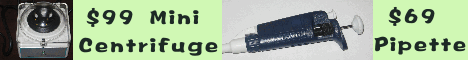Online | Download | Store | Contact | About

 Math/Stat Calculator           Click here for more molecular biology tools.
 Applet runs on Java-enabled browsers PC: Internet Explorer 5.5 or higher and Netscape 4.08 or higher Mac: Internet Explorer 5.0, IE5.1.4 or higher (IE5.1 will not work because of a bug.) Need to design a better siRNA? Please use SVM RNAi 3.6 for rational siRNA design.
 The Electronic Protocol Book Table of contents BioToolKit 300 Download Trials An electronic protocol book with 500 protocols and 100 recipes. A great quick and practical reference for bench scientists as well as for new students.   Get A Copy A collection of tools frequently used by bench biomedical scientists, ranging from centrifugation force conversion, molecular weight, OD, recipe calculators, to clinical calculators. Include all Primo 3.4, Abie 3.0, Heatmap Viewer, MicroHelper, Godlist Manager, label printing, and grade book.   More infoMathematics and Statistics Calculator The Math/Stat calculator is designed to work with columns of data. In general the data is in the following format: Input data format For functions with two varibles (+, -, *, and /), the input data should be in two columns as (x1, x2). The result of the operations are x1 + x2, x1 - x2, x1 * x2, and x1 / x2, respectively. For functions with one varible (i.e., cos( x ) ), the input data can be in one column or multiple columns. Results are displayed in a matrix form if the input data is in a matrix form. For funtions with array variable (i.e, max( x ), std( x )), the input data in each column is an array. Functions +   plus: x1 + x2 -   minus: x1 - x2 *   time: x1 * x2 /   divide: x1 / x2 abs   absolute value: |x| acos   arc cosine: acos(x) asin    arc sine: asin(x) atan    arc tangent: atan(x) atan2(x2, x1)    converts rectangular coordinates (x1, x2) to polar (r, theta) ceil    the smallest integer value that is not less than x: ceil(x) cos    the trigonometric cosine: cos(x) E    Euler's number e exp    Exponential function: exp(x) floor   the largest integer value that is not greater than x: floor(x) inverse   inverse: 1/x mod(x, a)    the remainder of x - n * a ln    the natural logarithm (base e): loge(x) log10    the logarithm (base 10): log10(x) loga    the logarithm (base a): loga(x) max    the greatest value in an array: max(x) mean    mean value of an array: mean(x) min    the smallest value in an array: min(x) PI    the constant Pi power    x to the a-th power: xa random    random number between 0 and 1 round    round to the coloses integer: round(x) round2    round to the a-th decimal point: round(x, a) std    standard deviation of an array: std(x) stdN-1    bias-corrected standard deviation of an array: stdN-1(x) sin    the trigonometric sine: sin(x) sqrt    positive square root: sqrt(x) sum    sum of an array: sum(x) tan    the trigonometric tangent: tan(x) toDegrees    converts an angle measured in radians to degrees toRadians    converts an angle measured in degrees to radians var    variance of an array: var(x) Thanks for using our software! Please let us know your suggestions and comments.
THIS SOFTWARE IS PROVIDED BY CHANG BIOSCIENCE ``AS IS'' AND ANY EXPRESS OR IMPLIED WARRANTIES, INCLUDING, BUT NOT LIMITED TO, THE IMPLIED WARRANTIES OF MERCHANTABILITY AND FITNESS FOR A PARTICULAR PURPOSE ARE DISCLAIMED. IN NO EVENT SHALL CHANG BIOSCIENCE OR ITS SPONSORS BE LIABLE FOR ANY DIRECT, INDIRECT, INCIDENTAL, SPECIAL, EXEMPLARY, OR CONSEQUENTIAL DAMAGES (INCLUDING, BUT NOT LIMITED TO, PROCUREMENT OF SUBSTITUTE GOODS OR SERVICES; LOSS OF USE, DATA, OR PROFITS; OR BUSINESS INTERRUPTION) HOWEVER CAUSED AND ON ANY THEORY OF LIABILITY, WHETHER IN CONTRACT, STRICT LIABILITY, OR TORT (INCLUDING NEGLIGENCE OR OTHERWISE) ARISING IN ANY WAY OUT OF THE USE OF THIS SOFTWARE, EVEN IF ADVISED OF THE POSSIBILITY OF SUCH DAMAGE.

Copyright © 2002-2004 Chang Bioscience, Inc. All rights reserved.